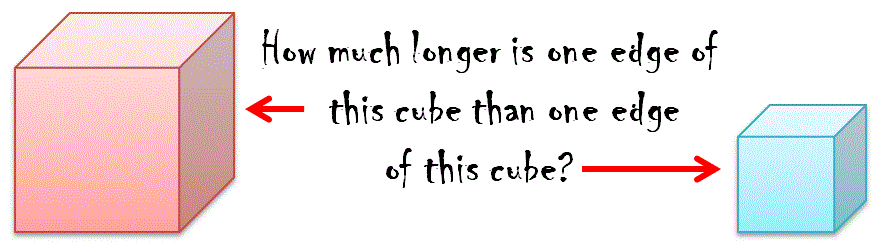#Volume = 8 cm3

Volume = 5832 mm3

DIAGRAM NOT TO SCALE

## A Mathematics Lesson Starter Of The Day

Hint: If you don't have a calculator with a cube root function here are the keys
you would need to press to find the cube root of 5832:

5832^(1÷3)

This works because raising a number to the power one third is equivalent to finding the cube root of the number.

Note to teacher: Doing this activity once with a class helps students develop strategies. It is only when they do this activity a second time that they will have the opportunity to practise those strategies. That is when the learning is consolidated. Click the button above to regenerate another version of this starter from random numbers.

Your access to the majority of the Transum resources continues to be free but you can help support the continued growth of the website by doing your Amazon shopping using the links on this page. Below is an Amazon link. As an Amazon Associate I earn a small amount from qualifying purchases which helps pay for the upkeep of this website.

Educational Technology on Amazon

Here is the URL which will take pupils to a self marking quiz about volume.

Transum.org/go/?to=Volume## Interesting fact for the Teacher!

There is a simple method to compute the cube roots using a non-scientific calculator, which requires only the multiplication and square root buttons. No memory is required. The following method is used:

• Press the square root button twice.
• Press the multiplication button.
• Press the square root button twice.
• Press the multiplication button.
• Press the square root button four times.
• Press the multiplication button.
• Press the square root button eight times.
• Press the multiplication button...

This process is continued until the number does not change when the multiplication button is pressed, since the repeated square root gives 1 (this means that the solution has been determined to as many significant digits as the calculator can handle). Then, press the square root button one last time. At this point an approximation of the cube root of the original number will be shown in the display.

A full explanation of why this works can be found onWikipedia, the free online encyclopedia.

## Curriculum Reference

See the National Curriculum page for links to related online activities and resources.

Transum,

Monday, August 12, 2013

"There are a number of concepts involved in this problem. The one most students find quite difficult to understand is converting cubic millimetres to cubic centimetres (or vice versa). Finding the cube root of a number is also challenge to most pupils. This is probably a starter that will require a certain amount of guidance, help and support from the teacher but once the method has been established refresh this page to get the same problem with different numbers."

JD, WA

Tuesday, August 12, 2014

"Students don't need to convert cubic mm to cubic cm. They can find the cube root of each and then they are converting mm to cm which is much easier!
"If there is a better solution find it!" - Thomas Eddison.

[Transum: Excellent observation JD. Thanks very much for sharing.]"

Do you have any comments? It is always useful to receive feedback and helps make this free resource even more useful for those learning Mathematics anywhere in the world. Click here to enter your comments.For All: